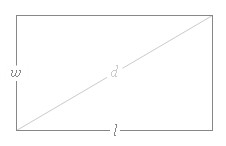## Rectangle Area & Diagonal Length Calculator

 Length of Rectangle Width of Rectangle Unit FeetInchesMeterCenti MeterMilli Meter
 Result: Rectangle Diagonal Length Perimeter of Rectangle Rectangle Surfacearea

In Geometry, rectangular is a shape with four straight sides, four angles of 90-degrees, and two of which are longer than the other two.

Rectangle Calculator to find out the Rectangle Diagonal Length, Perimeter of Rectangle and Rectangle Surface area.

### Rectangle Area & Diagonal Length formulaRectangle Area = Length x Width

Rectangle Perimeter = 2L + 2W

Rectangle Diagonal Length = Root of (L2 + W2)

Thinkcalculator.com provides you helpful and handy calculator resources.